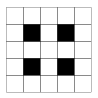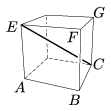Mathematical and Physical Journal
for High Schools
Issued by the MATFUND Foundation
 Already signed up? New to KöMaL?

# KöMaL Problems in Mathematics, March 2011

Show/hide problems of signs:## Problems with sign 'K'

Deadline expired on April 11, 2011.

K. 289. If the middle digit of a three-digit number is deleted, the resulting number is one seventh of the original number. Which three-digit number is it?

(6 pont)

solution (in Hungarian), statistics

K. 290. On my wife's birthday cake, her age was written in two digits, made of almond paste. We noticed that the same two digits would be suitable for my birthday cake, too, but they should then form a power expression. Given that the difference between our ages is equal to the sum of the two digits, find our ages.

(6 pont)

solution (in Hungarian), statistics

K. 291. 125 small cubes, 1 cm on edge, are glued together to form one big solid cube. Then holes of square cross section are bored through the cube, perpendicular to its faces. As a result, each face of the cube is as shown in the diagram (black squares indicating the position of the holes.) The resulting solid is dipped in red paint.

a) Find the volume of the resulting solid in cm3.

b) Find the total area in cm2 that is painted red.(6 pont)

solution (in Hungarian), statistics

K. 292. Consider the points A(0,0), B(b,2), C(b,5), D(0,d) on the coordinate plane. Given that the points form a trapezium ABCD of area 25 units, and that b and d are positive integers, find the values of the missing coordinates of the vertices.

(6 pont)

solution (in Hungarian), statistics

K. 293. Fred's cows are grazing on a field. Each cow eats the same daily amount of grass, independently of the actual number of cows on the field. One day, Fred took 6 cows to the field. It took the cows 3 days to eat all the grass on the field, so at the end of the third day Fred had to withdraw his cows from the field to let the grass grow back. When the original amount of grass was restored, Fred took 3 cows to the field. He was surprised to observe that this time it took 7 days for the cows to eat up all the grass. He was so puzzled that he asked Ben who was good at maths. Ben reminded him that he had forgotten about something while watching the cows graze and counting the days. What did Fred forget, and how long would it take a single cow to eat all the grass in the field?

(6 pont)

solution (in Hungarian), statistics

K. 294. Find at least two words (in any language, meaningful strings of letters) of different lengths, such that the letters can be ordered in exactly 20 different ways. Identical letters are not distinguished.

(6 pont)

solution (in Hungarian), statistics## Problems with sign 'C'

Deadline expired on April 11, 2011.

C. 1070. In base-n notation, a number has the form 2011, and in base-(n+3) notation it is 537. Which number is it?

(5 pont)

solution (in Hungarian), statistics

C. 1071. The forum of this journal on the internet has exactly 1000 registered members. Each member sends an e-mail to one other member. Prove that it is possible to select a group of 334 members such that within the group no one sent an e-mail to anyone else.

(5 pont)

solution (in Hungarian), statistics

C. 1072. Prove that the radius of the inscribed circle of a right-angled triangle is smaller than three tenths of the longer leg.

(5 pont)

solution (in Hungarian), statistics

C. 1073. The function f(x)=x3+ax2+bx+c () has at least two zeros. Prove that a2>3b.

(5 pont)

solution (in Hungarian), statistics

C. 1074. Find the distance between edge AB of a unit cube and diagonal EC of the cube (see the figure).(5 pont)

solution (in Hungarian), statistics## Problems with sign 'B'

Deadline expired on May 10, 2011.

B. 4342. In the lottery, 5 numbers are drawn out of the numbers 1 to 90. If the numbers drawn are always listed in increasing order, which number will occur the most frequently in the second place?

(3 pont)

solution (in Hungarian), statistics

B. 4343. Let a and b denote positive numbers such that a3+b3=1. Show that a2+ab+b2-a-b>0.

(Suggested by J. Pataki, Budapest)

(4 pont)

solution (in Hungarian), statistics

B. 4344. The lengths of the parallel sides of a symmetric trapezium are a and c. The midpoints of the legs are E and F. Let G denote the orthogonal projection of point E on the line of the leg BC. What is the area of the trapezium if point C divides the line segment GF in a 1:2 ratio? (C lies closer to point G.)

(4 pont)

solution (in Hungarian), statistics

B. 4345. Prove that in any set of seven different positive integers there are three numbers such that the greatest common divisor of any two of them leaves the same remainder when divided by three.

(Suggested by S. Kiss, Budapest)

(4 pont)

solution (in Hungarian), statistics

B. 4346. Given a convex pentagon, construct a line that halves its area.

(Suggested by T. Horváth, (Dunakeszi)

(3 pont)

solution (in Hungarian), statistics

B. 4347. A rectangle is divided into at least two squares of different size. Prove that it is not possible for the lengths of the sides of all squares to be Fibonacci numbers.

(Suggested by G. Damásdi, (Kecskemét)

(4 pont)

solution (in Hungarian), statistics

B. 4348. The diagonals of a cyclic quadrilateral ABCD are not perpendicular. The feet of the perpendiculars dropped from the vertices A, B, C, D onto the diagonals not passing through them are A', B', C', D', respectively. The intersections of lines AA' and DD', DD' and CC', CC' and BB', and finally, BB' and AA' are E, F, G, H, respectively. Prove that A'B'C'D' is a cyclic quadrilateral with the centre of its circumscribed circle lying at the intersection of the line segments EG and FH.

(Suggested by B. Bíró, (Eger)

(5 pont)

solution (in Hungarian), statistics

B. 4349. Prove that if a quadrilateral can be covered with a disc of unit radius then the product of its sides cannot be more than 4.

(4 pont)

solution (in Hungarian), statistics

B. 4350. In a tetrahedron A1A2A3A4, for every interior point P and for any order i, j, k, l of the numbers 1, 2, 3, 4, the following inequality is true: PAi+PAj+PAk<AlAi+AlAj+AlAk. Does it follow that the tetrahedron is regular?

(5 pont)

solution (in Hungarian), statistics

B. 4351. Some subsets containing at least two elements of an n-element set are selected. Given that any two elements of the set occur together exactly once in some selected subset, prove that the number of the subsets selected is either 1 or at least n.

(5 pont)

solution (in Hungarian), statistics## Problems with sign 'A'

Deadline expired on April 11, 2011.

A. 530. There are given 2n+1 points(n2) lying on a circle in this order such that. The linessplit the line segment A1A2n+1 into 2n-1 part; denote their lengths by, respectively. Prove that.

(Proposed by Zoltán Gyenes, Budapest)

(5 pont)

statistics

A. 531. Prove that for every positive integer k there is a positive integer Nk (depending only on k) such that wheneveris a set system whose elements are at most k-element sets such that every two elements ofhave an element in common, then there exists a set A with at most Nk elements such that every two elements ofand A have an element in common.

(Proposed by: Ambrus Zsbán, Budapest)

(5 pont)

statistics

A. 532. Prove that there exist a real number c>0 such that whenever each of the numbersare 1 or -1 and the polynomial (x-1)k divides the polynomialthen k<c.ln2(n+1).

(5 pont)

solution (in Hungarian), statistics